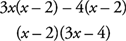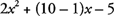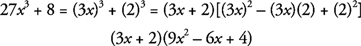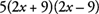## Factoring Polynomials

Factoring, the process of “unmultiplying” polynomials in order to return to a unique string of polynomials of lesser degree whose product is the original polynomial, is the simplest way to solve equations of higher degree. Although you should already be proficient in factoring, here are the methods you should be familiar with, in case you need to reviews.

## Greatest common factors

If all of the terms in a polynomial contain one or more identical factors, combine those similar factors into one monomial, called the greatest common factor, and rewrite the polynomial in factored form.

Example 1: Factor the expressions.

• (a)

15 x 3 + 5 x 2 −25 x

Since each term in the polynomial is divisible by both x and 5, the greatest common factor is 5 x. In factored form, the polynomial is written 5 x(3 x 2 + x − 5).

• (b)

18 x 3 y 5 z 4 + 6 x 2 yz 3 − 9 x 2 y 3 z 2

The largest monomial by which each of the terms is evenly divisible, thus the greatest common factor, is 3 x 2 yz 2, so factor it out.## Factoring by grouping

Sometimes, the greatest common factor of an expression is not just a monomial but an entire parenthetical quantity. You are allowed to factor out quantities in parentheses just as you can factor out individual terms.

Example 2: Factor the following expressions.

• (a)

3 x( x − 5) + 2 y( x − 5) − 10( x − 5)

The only thing that divides into each of these terms evenly is the linear expression ( x − 5). Factor it out, just as you would any greatest common factor, leaving behind the monomial in each term that was multiplied by ( x − 5):• (b)

3 x 2 − 6 x − 4 x + 8

Nothing, except the number 1, divides evenly into each of the terms, and there's no use factoring out 1. However, the first two terms have a greatest common factor of 3 x. Furthermore, if you factor −4 out of the final two terms, you can factor by grouping:## Factoring quadratic trinomials

Your most common factoring task, aside from greatest common factoring, is changing a quadratic trinomial into the product of two linear binomials.

Example 3: Factor the following expressions.

• (a)

x 2 − 4 x − 12

If the leading coefficient is 1, as it is here, the process is simple. Find two numbers whose sum equals the coefficient of the x term and whose product is equal to the constant term. The only two numbers whose sum is −4 and that multiply to give −12 are −6 and 2. Use these as the constants in the linear factors:• (b)

x 2 − 10 x + 24

Since this quadratic trinomial has a leading coefficient of 1, find two numbers with a product of 24 and a sum of −10. Through some experimenting, you'll find those numbers are −6 and −4:• (c)

2 x 2 + 9 x − 5

If the leading coefficient is not 1, you must follow another procedure. You still seek two numbers, and those numbers will still add up to 9. However, they will multiply to give you −10, the product of the leading coefficient and the constant. (You didn't have to use this technique when the leading coefficient was 1, since the product of the leading coefficient and the constant would just have been the constant anyway.) The numbers in question are 10 and −1. Rewrite the x coefficient as the sum of those numbers:Distribute the x to both 10 and −1:To finish, factor by grouping:## Special factor patterns

Occasionally, the only effort you'll have to expend on a factoring problem is recognizing that the polynomial in question matches one of three specific patterns. You should memorize each of these formulas so that you can spot them instantly:

• Difference of perfect squares: x 2a 2 = ( x + a)( xa)
• Difference of perfect cubes: a 3b 3 = ( axs − b)( a 2 + ab + b 2)
• Sum of perfect cubes: a 3 + b 3 = ( a + b)( a 2ab + b 2)

Example 4: Factor the following expressions completely.

• (a)

27 x 3 + 8

Note that 27 x 3and 8 are both perfect cubes, so apply the sum of perfect cubes formula:• (b)

20 x 2 − 405

This doesn't appear to match any of the patterns, but you can factor out a greatest common factor of 5 to begin:Now it's clearer that (4 x 2 − 81) is the difference of perfect squares, since (2 x) 2 = 4 x 2and 9 2 = 81:Back to Top
A18ACD436D5A3997E3DA2573E3FD792A